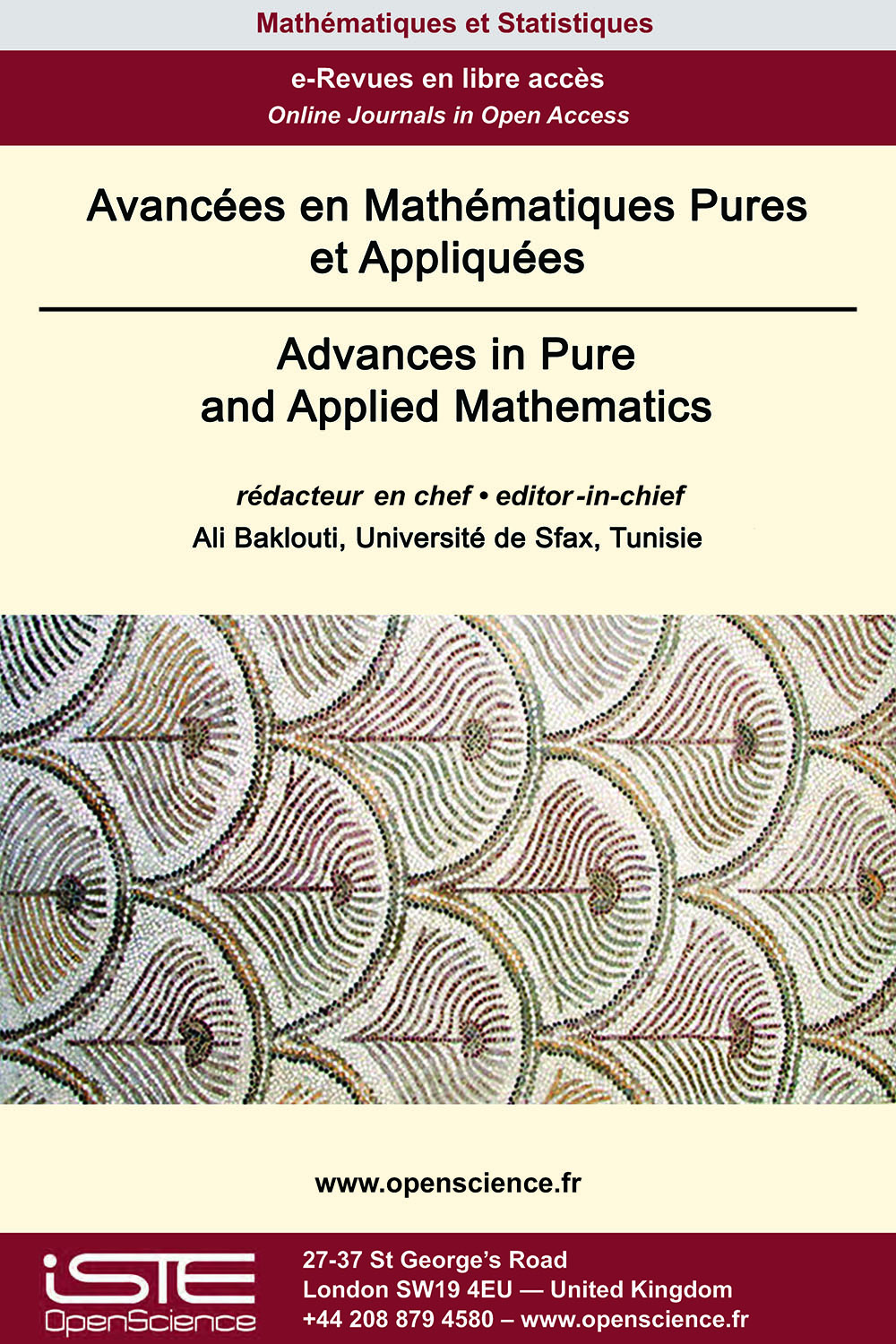# Vol 12 - Numéro 2 (Mai 2021)

## Articles parus

Approximation des fonctions à valeur complexe par les fonctions fractales

Fractal approximants developed through iterated function systems (IFS) prove more versatile than classical approximants. In this paper, we introduce a new class of fractal approximants using the suitable bounded linear operators defined on the space C(I) of continuous functions and concept of $\alpha$-fractal functions. The convergence of the proposed fractal approximants towards the original continuous function does not need any condition on the scaling factors. The fractal approximants proposed in this paper possess fractality and convergence simultaneously. Without imposing any condition on the scaling vector, we establish the constrained approximation by the proposed fractal approximants. Existence of Schauder basis of fractal polynomials for the space of continuous functions C(I) is investigated. Using the proposed class of fractal approximants, we develop complex fractal approximants for representation of the square integrable complex-valued functions defined on a real compact interval.

Estimations à priori pour l’équation elliptique super-linéaire : le problème de la valeur au bord de Neumann

$\mbox{In this paper we study the nonexistence of finite Morse index solutions of the following Neumann boundary value problems}\\ {(Eq.H)} \begin{cases} -\Delta u = (u^{+})^{p} \;\; \text{in  \mathbb{R}_+^N}, \\ \frac{\partial u}{\partial x_{N}}=0 \quad\quad\;\; \text{ on  \partial\mathbb{R}_+^N}, \\ u \in C^2(\overline{\mathbb{R}_+^N}) \mbox{ and sign-changing, }\\u^+ \mbox{ is bounded and } i(u)<\infty,\end{cases}\\ \mbox{or}\\ {(Eq.H')}\begin{cases}-\Delta u = |u|^{p-1}u \text{ in  \mathbb{R}_+^N}, \\ \frac{\partial u}{\partial x_{N}}=0 \;\;\;\;\;\;\;\;\quad\text{ on  \partial\mathbb{R}_+^N}, \\ u \in C^2(\overline{\mathbb{R}_+^N}),\\ u \mbox{ is bounded and } i(u) < \infty.\end{cases}\\ \mbox{ As a consequence, we establish the relevant Bahri-Lions's }L^\infty\mbox{-estimate  via the boundedness of Morse index of solutions to}\\ \begin{equation}\label{1.1} \left\{\begin{array}{lll} -\Delta u=f(x,u) &\text{in  \Omega,}\\ \frac{\partial u}{\partial \nu}=0 &\text{on \partial \Omega,} \end{array} \right. \end{equation}\\ \mbox{where} f \mbox{ has an asymptotical behavior at in-nity} \mbox{which is not necessarily the same at} \pm\infty. \mbox{Our results complete previous Liouville}\\ \mbox{ type theorems and } L^\infty\mbox{-bounds via Morse index obtained in [3, 6, 13, 16, 12, 21].}$

Les opérateurs maximaux dans les espaces de Dunkle-Fofana

We generalize Wiener amalgam spaces by using Dunkl translation instead of the classical one, and we give some relationship between these spaces, Dunkl-Lebesgue spaces and Dunkl-Morrey spaces. We prove that the Hardy-Litlewood maximal function associated with the Dunkl operators is bounded on these generalized Dunkl-Morrey spaces.

Sur le contrôle d’un système non-linéaire d’équations viscoélastiques

In this paper we consider a nonlinear system of two coupled viscoelastic equations, prove the well posedness, and investigate the asymptotic behaviour of this system. We use minimal and general conditions on the relaxation functions and establish explicit energy decay formula which gives the best decay rates expected under this level of generality. Our new result generalizes the earlier related results in the literature.### Autres numéros :

2020

Volume 20- 11

Numéro 1 (Mai 2020)
Numéro 2 (Septembre 2020)

2021

Volume 21- 12

Numéro 1 (Janvier 2021)
Numéro 2 (Mai 2021)
Numéro spécial : AUS-ICMS 2020
Numéro 3 (Septembre 2021)

2022

Volume 22- 13

À paraître

Numéro 1 (Janvier 2022)
Numéro 2 (Mars 2022)